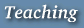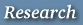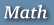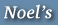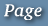# Math 6843     Geometric Group Theory         Spring 2000

## Information and Messages

• The Math 6843 course announcement.
• Office hours are by appointment or email.
• List of topics. Current one is highlighted.
• Infinite groups as geometric objects.
• Word metric, Cayley graph, examples
• Quasi isometry, geodesic spaces, path metric, Hopf Rinow theorem, Milnor theorem
• Statement of some q.i. classification and rigidity results
• some q.i. invariants in group theory
• Hyperbolic groups.
• Motivation/Background:
- word problem in free groups
- Dehn's algorithm for closed surface groups
- basic geometry of hyperbolic plane and hyperbolic 3-space
• Delta-hyperbolic metric spaces:
- various definitions
- divergence of geodesics
- intro to the boundary
• Hyperbolic groups:
- various defintions
- IP inequalities, Dehn's algorithm
- basic properties
• CAT(0) and CAT(-1) spaces and groups.
• Definitions and basic properties
• Constructing CAT(0) groups